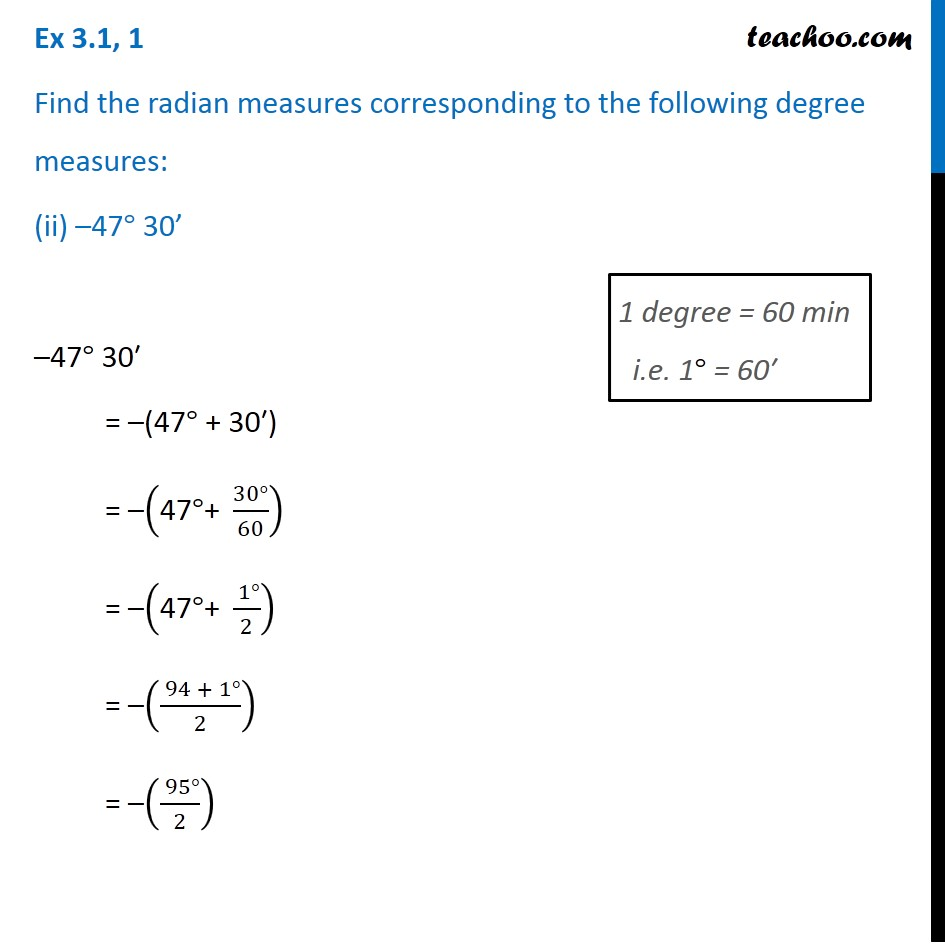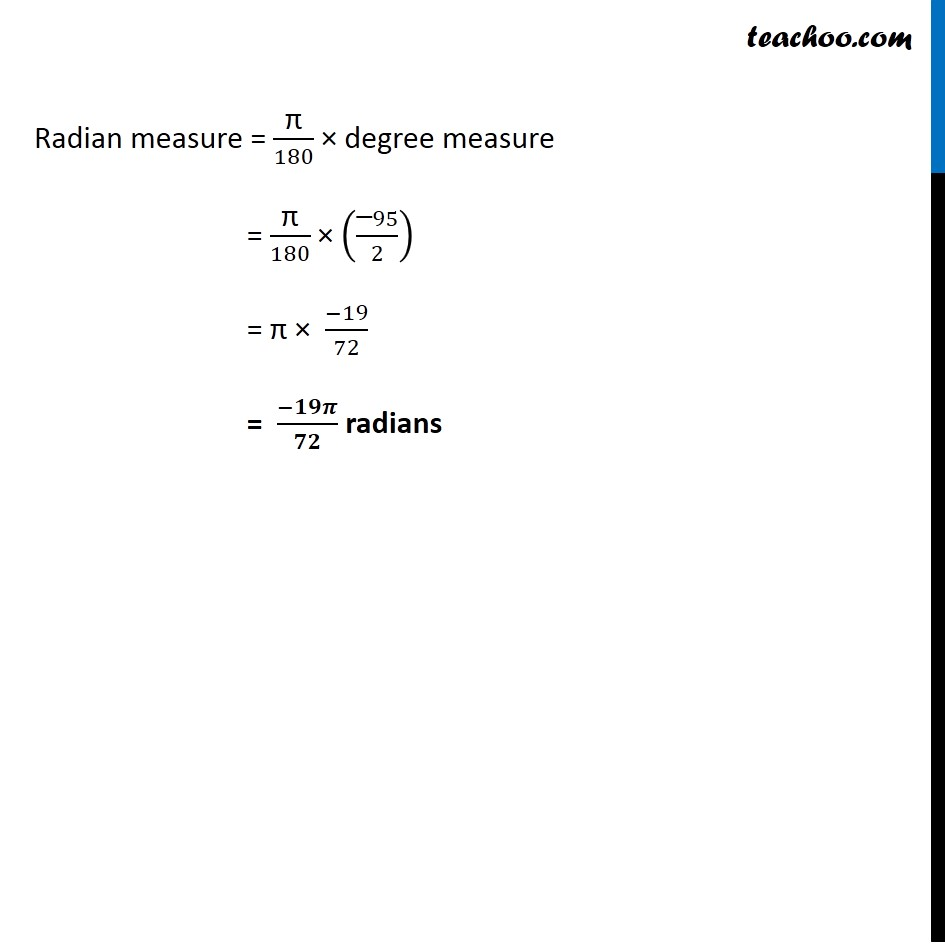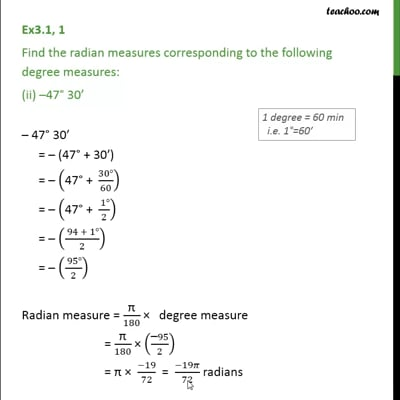Ex 3.1

Chapter 3 Class 11 Trigonometric Functions
Serial order wiseThis video is only available for Teachoo black users

Maths Crash Course - Live lectures + all videos + Real time Doubt solving!

### Transcript

Ex 3.1, 1 Find the radian measures corresponding to the following degree measures: (ii) –47° 30’ –47° 30′ = –(47° + 30′) = –("47" °"+ " (30°)/60) = –("47" °"+ " ( 1°)/2) = –(( 94 + 1°)/2) = –(( 95°)/2) 1 degree = 60 min i.e. 1° = 60′ Radian measure = "π" /180 × degree measure = "π" /180 × (("–" 95)/2) = "π" × (−19)/72 = (−𝟏𝟗𝝅)/𝟕𝟐 radians